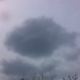计算几何学习笔记

向量

1.四则运算

• 加法：横纵坐标分别相加
• 减法：横纵坐标分别相减
• 数乘：横纵坐标分别乘以乘数
• 除法：横纵坐标分别除以除数
• 相等：横纵左边分别相等

2.旋转$sin(a+b)=sina*cosb+sinb*cosa$

$cos(a+b)=cosa*cosb-sina*sinb$

3.点积

$$a \cdot b$$的集合意义为a在b上的投影长度乘以b的长度

$$a \cdot b=|a||b|cos<a,b>$$

$a=(x_1,y_1),b=(x_2,y_2),a \cdot b=(x_1 * x_2,y_1 * y_2)$$$V_1 \cdot V_2=h \times |V1|$$

$$\because$$    $$V_1=\frac{y_1}{\cos \alpha}\,V_2=\frac{y_2}{\cos \beta}$$

$$\therefore$$    $$V_1 \cdot V_2=\frac{y_2}{\cos \beta } \times \cos (\beta – \alpha ) \times \frac{y_1}{\cos \alpha }$$

$$V_1 \cdot V_2=\frac{y_1y_2}{\cos \beta \cos \alpha } \times \cos (\beta – \alpha )$$

$$\because$$    $$\cos (\beta – \alpha )= \cos \alpha \cos \beta + \sin \alpha \sin \beta$$

$$\therefore$$    $$V_1 \cdot V_2=y_1y_2 + \sin \alpha \sin \beta \frac{y_1y_2}{\cos \beta \cos \alpha }$$

$$\because$$    $$\frac{\sin \alpha }{\cos \alpha }=\tan \alpha$$

$$\therefore$$    $$V_1 \cdot V_2=y_1y_2 + \tan \alpha \tan \beta y_1y_2$$

$$\therefore$$    $$V_1 \cdot V_2=y_1y_2 + x_1x_2$$

$$证毕$$

• 点积满足交换律
• 两个向量夹角是一个锐角时，点积为正数；为直角时点积为零（因此可用点积判垂直）；为钝角时点积为负数

4.叉积

$$a \times b$$的几何意义就是ab所形成的平行四边形的有向面积。

$a=(x_1,y_1),b=(x_2,y_2),a \times b=x_1 * y_2 – x_2 * y_1$poj 2318
poj 2398

5.单位向量与单位法向量

$$\frac{a}{|a|}$$为a的单位向量，方向相同，长度为1

6.判断向量关系点与直线

poj 1269

2.直线交点

$$t_1=\frac{cross(w,u)}{cross(v,w)},t_2=\frac{cross(v,u)}{cross(v,w)}$$

5.点到线段的距离poj 1269

poj 2653
poj 2826
poj 1410

poj 1654

poj 1584

凸包

2.计算流程

1. 将点按x坐标第一关键字，y坐标第二关键字排序
2. 首先将p1,p2压入栈中
3. 判断下一个点和栈中最后一个点组成的向量是否在当前前进方向的左边（求下凸壳），如果是的话就入栈，否则弹栈
4. 这样求出了下凸壳，再倒着做一遍就求出来了正凸壳，合起来就是完整的凸包COGS 896
pku 1113
pku 1228
pku 1873
pku 3348

3.凸包应用：旋转卡壳1.2.Compute the polygon’s extreme points in the y direction. Call them ymin and ymax. Construct two horizontal lines of support through ymin and ymax. Since this is already an anti-podal pair, compute the distance, and keep as maximum. Rotate the lines until one is flush with an edge of the polygon. A new anti-podal pair is determined. Compute the new distance, compare to old maximum, and update if necessary. Repeat steps 3 and 4 until the anti-podal pair considered is (ymin,ymax) again. Output the pair(s) determining the maximum as the diameter pair(s).poj 2187
poj 2079
bzoj 1069
bzoj 1185

pku 3130
pku 1474
pku 1279
pku 3525
pku 3384

后记

1.slhippo2017-03-04 下午8:50

数型结合好典范。

2.Leaves2018-01-25 上午11:11

太强辣！
膜拜啊！

3.Idvz2018-03-07 下午5:11

叉积得到的是向量，应该不是标量吧…

•远航之曲2018-03-07 下午8:14

在计算几何里面应用的都是叉积的长度，就简略直接求成长度了。。

说的的确不大准确

×